# Digital Electronics - Boolean Algebra and Logic Simplification

### Exercise :: Boolean Algebra and Logic Simplification - General Questions

26.

The truth table for the SOP expression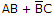has how many input combinations?

 A. 1 B. 2 C. 4 D. 8

Answer: Option D

Explanation:

No answer description available for this question. Let us discuss.

27.

Converting the Boolean expression LM + M(NO + PQ) to SOP form, we get ________.

 A. LM + MNOPQ B. L + MNO + MPQ C. LM + M + NO + MPQ D. LM + MNO + MPQ

Answer: Option D

Explanation:

No answer description available for this question. Let us discuss.

28.

A Karnaugh map is a systematic way of reducing which type of expression?

 A. product-of-sums B. exclusive NOR C. sum-of-products D. those with overbars

Answer: Option C

Explanation:

No answer description available for this question. Let us discuss.

29.

The Boolean expression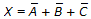is logically equivalent to what single gate?

 A. NAND B. NOR C. AND D. OR

Answer: Option A

Explanation:

No answer description available for this question. Let us discuss.

30.

Applying the distributive law to the expression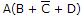, we get ________.

 A.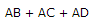B.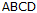C.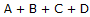D.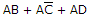Answer: Option D

Explanation:

No answer description available for this question. Let us discuss.

#### Current Affairs 2021

Interview Questions and Answers